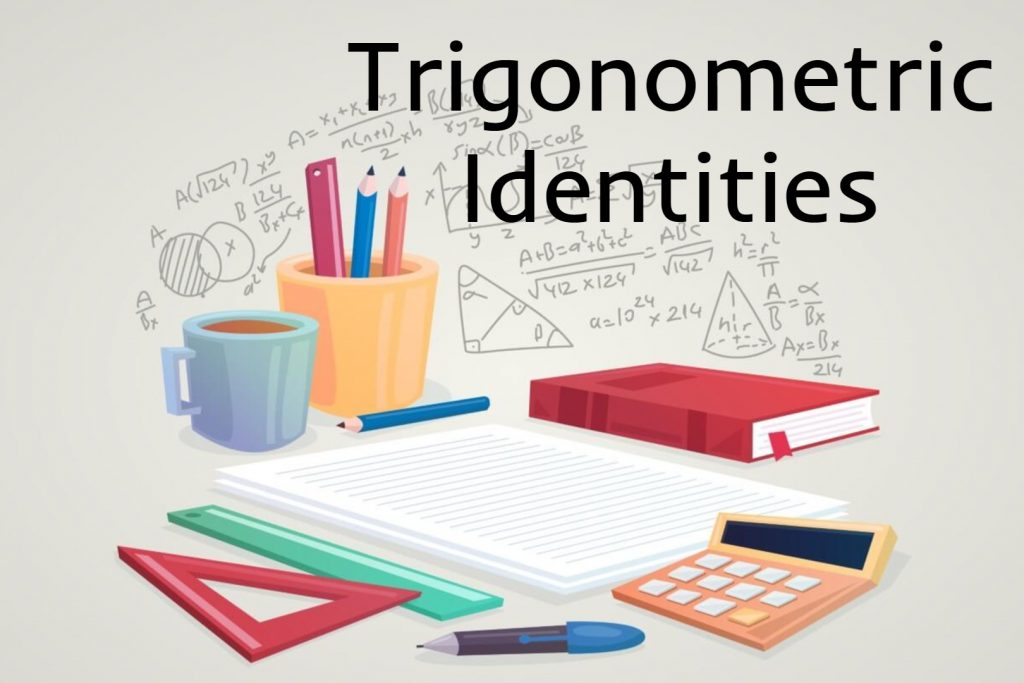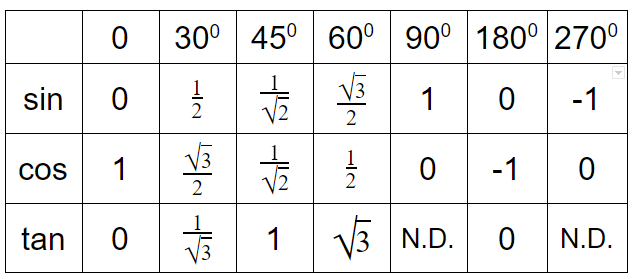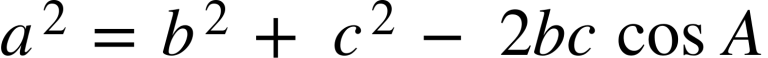# Trigonometric Identities

A Trigonometric identity or trig identity is an identity that contains the trigonometric functions sine(sin), cosine(cos), tangent(tan), cotangent(cot), secant(sec), or cosecant(csc). Trigonometric identities can use to:

• Simplify trigonometric expressions.
• Solve trigonometric equations.
• Prove that one trigonometric expression is equivalent to another so that we can replace the first expression by the second expression. The following expression can give us new insights into some applications that the first one doesn’t show.
• Quotient Identities
• Pythagorean Identities
• Trig Values Table
• Sum/Difference Formulas
• Double Angle Identities
• Sum to Product of Two Angles
• Product to Sum of Two Angles
• Law of sines
• Law of cosines
• Area of Triangle

## How Many Trigonometric Identities Available?

There is an infinite number of trig identities. Only a number of are useful, the rest generally ending up as problems and exercises for college students to unravel.

## Basic trigonometric identities fall into four groups:

• Reciprocals
• Ratios
• Products
• Pythagorean Theorem

### Quotient Identities### Pythagorean Identities### Trig Values Table*N.D. – Not Defined

### Sum/Difference Formulas### Double Angle Identities

2θcos 2θ3θ### Sum to Product of Two Angles### Product to Sum of Two Angles### Law of sines### Law of cosines### Area of Triangle1.2.